### IMO Shortlist 1961 problem 4

Kvaliteta:
Avg: 0.0
Težina:
Avg: 0.0
Consider triangle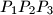$P_1P_2P_3$ and a point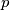$p$ within the triangle. Lines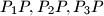$P_1P, P_2P, P_3P$ intersect the opposite sides in points$Q_1, Q_2, Q_3$ respectively. Prove that, of the numbers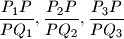at least one is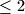$\leq 2$ and at least one is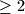$\geq 2$
Source: Međunarodna matematička olimpijada, shortlist 1961